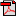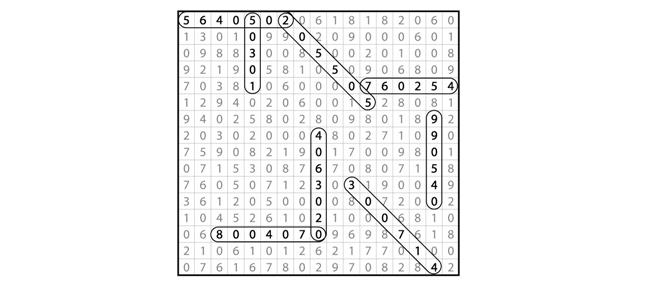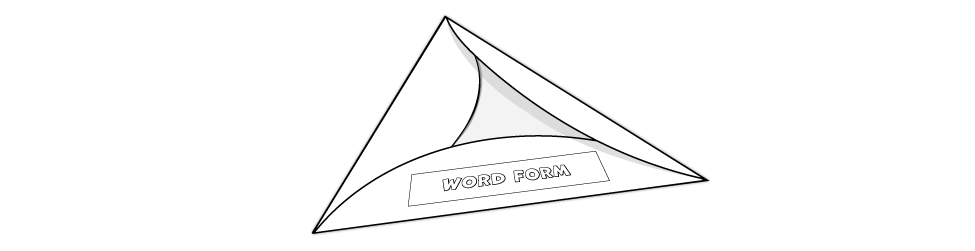# Lesson 1: Numbers to 1,000,000 Review

## Day 2

### Activity 3: Big Number Search

Use the clues provided on the "Big Number Search" sheet to find the numbers hidden in the puzzle. The numbers may be found horizontally, vertically, or diagonally, but the digits must be in the correct order from the millions to the ones places.Student Activity Page
This activity provides additional practice with recognizing numbers written in different forms.### Activity 4: The Place Is Right!

Complete the "Place is Right!" sheet by using the clues to figure out the numbers. Read through all of the clues before you try to figure out the number as many of the clues are connected to other clues, and you may need to use multiple clues to narrow down a particular digit. You may want to use a scratch sheet of paper to keep track of your options.

Keep in mind that a factor is a number that is multiplied by another number to get a product while a multiple is the product of a number and another number. For example, 6 is a factor of 12 because it can be multiplied by another number (2) to get 12. Twelve is a multiple of 2 and 3 because 2 can be multiplied by 6 and 3 can be multiplied by 4 to get 12.

Remember, too, that a prime number is a number greater than 1 that has only 2 factors, the number itself and the number 1 (for example, 2, 3, 11). A composite number is a number that has more than 2 factors (for example, 4, 6, 12). The number 1 is neither prime nor composite. Is 15 prime or composite? What about 13? You should see that 15 is composite because 1 times 15 and 3 times 5 both equal 15 and 13 is prime because its only factors are 1 and 13.

When you've finished the "Place if Right!" sheet, ask a family member or a friend to try to figure out the number that your own clues describe.Student Activity Page
During this activity, your child will use clues to figure out two big numbers — 8,532,241 and 5,351,799. Many of the clues are connected to one another, so as needed, remind your child to read the clues carefully.

To be successful with this activity, your child will review these facts about numbers:
• A factor is a number that is multiplied by another number to get a product (for example, 6 is a factor of 12).
• A multiple is the product of a number and another number (for example, 6 is a multiple of 2 and 3).
• A prime number is a number greater than 1 that has only 2 factors, the number itself and 1 (for example, 2, 3, 11).
• A composite number is a number that has more than 2 factors (for example, 4, 6, 12).
When your child finishes the "Place is Right!" sheet, allow him to share the clues he wrote on the back of the sheet so that someone else can try to figure out the number he has described.

### Activity 5: Big Numbers All Around!

Materials: 3-hole drilled card stock (kit), colored pencils, glue or glue stick, Interactive Notebook, scissors
1. Find the "Number Forms" page and cut out the circle and boxes along the dotted lines.
2. Fold the circle along the solid lines to cover the triangle. (Refer to the following image as needed.)
3. Glue each box with the form name to the top of the circle section that has the matching form underneath.
4. Use the forms on the circle to write the number form of the number inside the triangle. Use a red pencil to write commas in the correct places.
5. Add hyphens to the word form where they go using a blue pencil.
6. The addition signs are missing in the expanded form. Use a green pencil to write where they belong.
7. Use an orange pencil to draw the parentheses around each place value group in the expanded notation section.
8. Glue the template onto a piece of card stock, and place it in your Interactive Notebook as a helpful reference tool.Next, use a reference book or, with your parent's permission, the Internet to find a fact that involves a big number. Some examples might be the population of New York City, the distance from the Earth to the moon, or the number of hours in a century. Write your number in the box at the top of the activity sheet "Big Numbers All Around!" Then follow the directions in the six activity boxes to analyze your number.Student Activity PageStudent Activity Page
Your child will first create a foldable for his Interactive Notebook to remind him about the different forms that can be used to show a number. After cutting out and folding the circle, he should paste the correct form name to each outside flap, and then write the number form of the number on the the side of the triangle under the flap. He will finalize his foldable by adding hyphens, plus signs, or parentheses to the number forms in which they belong. Make sure the number forms are accurate and have commas in the correct places.
• Word Form: 2,438,096 — two million four hundred thirty-eight thousand ninety-six (the word form should have hyphens for "thirty-eight" and "ninety-six" and no commas)
• Expanded Form: 2,438,096 — 2,000,000 + 400,000 + 30,000 + 8,000 + 90 + 6 (your child should add the plus signs; note that expanded form does not include 0 values, so your child should not represent the 0 in the hundreds place because no valued number is included in that place)
• Expanded Notation: 2,438,096 — (2 × 1,000,000) + (4 × 100,000) + (3 × 10,000) + (8 × 1,000) + (0 × 100) + (9 × 10) + (6 × 1) (your child should insert the parentheses)
Next, your child will find a fact involving a big number. Some examples might be the population of New York City, the distance from the Earth to the moon, or the number of hours in a century. He will write that number at the top of the "Big Numbers All Around!" activity page and then analyze the number. Answers will vary depending on the number selected.